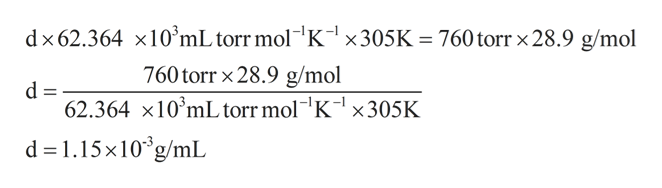# Determine the density of air at 32 degrees Celsius and 760 torr. The molar mass of air is 28.9 g/mol. Assume ideal behavior.

Question

Determine the density of air at 32 degrees Celsius and 760 torr. The molar mass of air is 28.9 g/mol. Assume ideal behavior.

check_circle

Step 1

As per the data given in the question,

Density of air, d = to be calculated

Temperature, T = 32C = 32 + 273 K = 305 K

Pressure of air, P = 760 torr

molar mass of air, M = 28.9 g/mol

Step 2

The relation between all the above parameters is for the gas showing ideal behavior is shown as follows:

Step 3

On substituting all the values in t...help_outlineImage Transcriptionclosedx62.364 x10'mL torr mol K1 x305K 760torr x 28.9 g/mol 760torr x28.9 g/mol d 62.364 x10 mL torr mol"K-1 X305K d 1.15x10g/mL fullscreen

### Want to see the full answer?

See Solution

#### Want to see this answer and more?

Solutions are written by subject experts who are available 24/7. Questions are typically answered within 1 hour.*

See Solution
*Response times may vary by subject and question.
Tagged in

### Chemistry